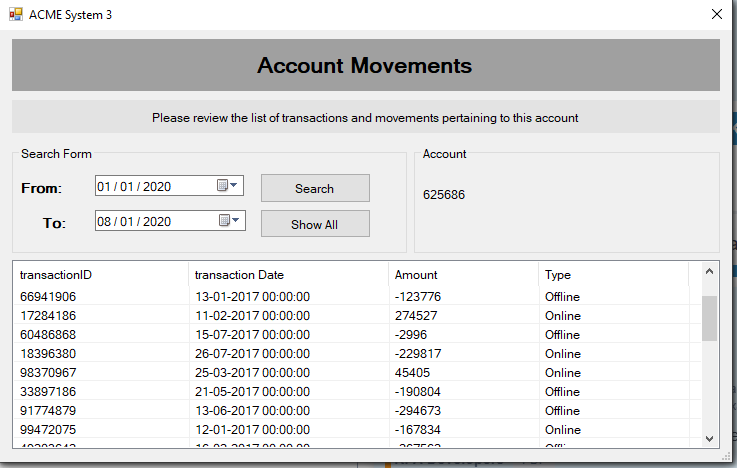# Calculate total amount

I want to calculate the total amount. so how can I use it?

2 Likes

Scraped the datat and pass the scraped to Datatable variable and use this

dataTable.AsEnumerable().Sum(Function(row) row.Field(Of Decimal)(“Amount”))

@Aarti_Godhasara

cheersHappy learning1 Like

If you are extracted the data from that table, then you can calculate the total amount using the below line of code.

``````yourTable.Compute("Sum(Amount)", String.Empty)
``````

it is not structure data.

I wan to get a total of amount but it is not structure dataAs others have pointed out: This looks like pretty structured data and you should be able to extract it with the Data Scraping wizard. Please don’t open duplicate topics like this.

Cheers

1 Like

Data scrapping is not supporting in this

Why not?

Do you have the option to export this table somehow?